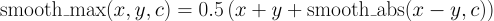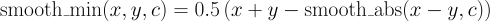# Smoothed Mathematical Functions¶

The functions in this section are smoothed equivalents of the original math functions that can be used to allow computing derivatives around discontinuities.

T smooth_abs(T x, T c = 0.001)

Smoothed version of abs(), defined as:Parameters:
T x

The input value

T c = 0.001

Cut-off point for the spline-approximated area (default: 0.001)

Returns:

The smoothed absolute value, defined as above

T smooth_max(T x, T y, T c = 0.001)

Smoothed version of the max(), defined as:Parameters:
T x

First argument to max

T y

Second argument to max

T c = 0.001

Cut-off point for the spline-approximated area (default: 0.001)

Returns:

The smoothed max function, defined as above

T smooth_min(T x, T y, T c = 0.001)

Smoothed version of the min(), defined as:Parameters:
T x

First argument to min

T y

Second argument to min

T c = 0.001

Cut-off point for the spline-approximated area (default: 0.001)

Returns:

The smoothed min function, defined as above

Last update: January 2023